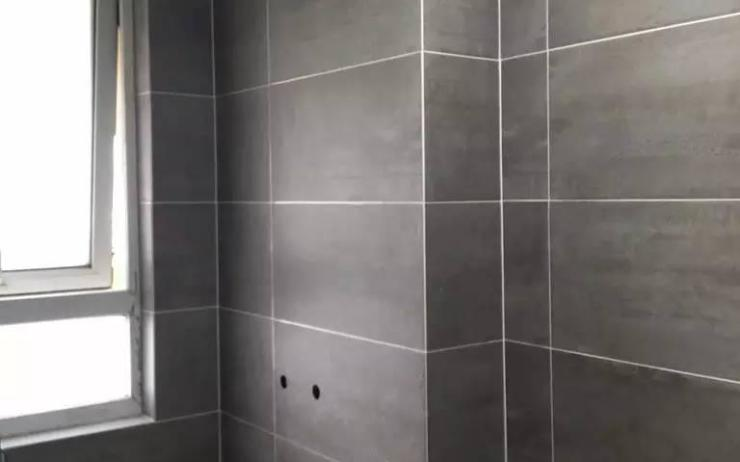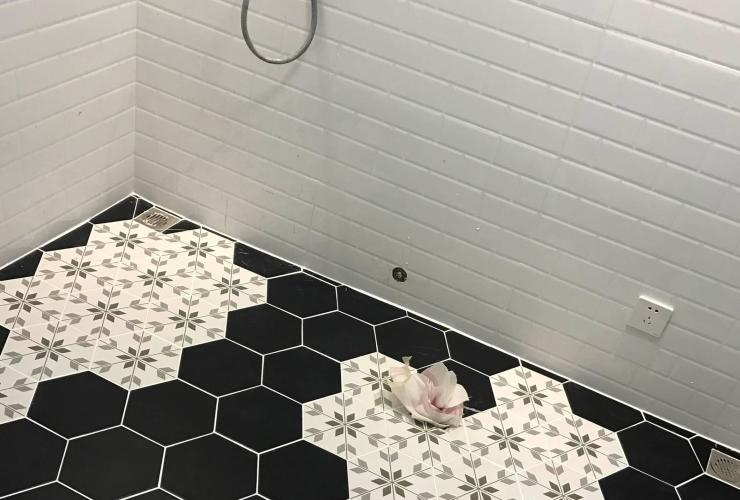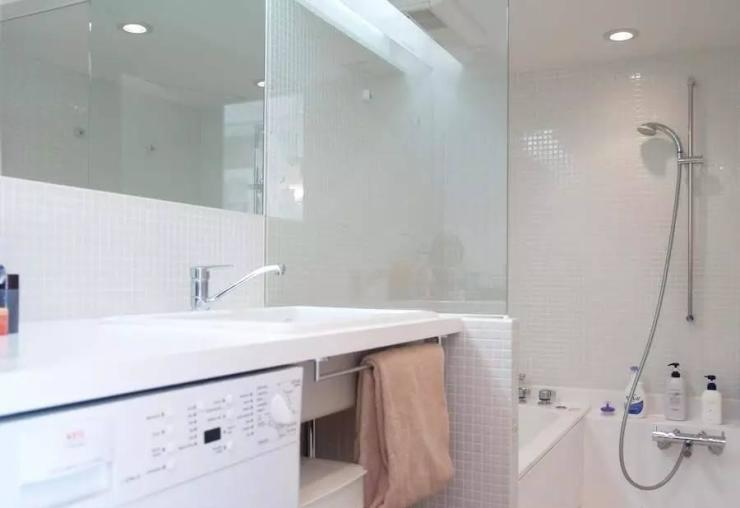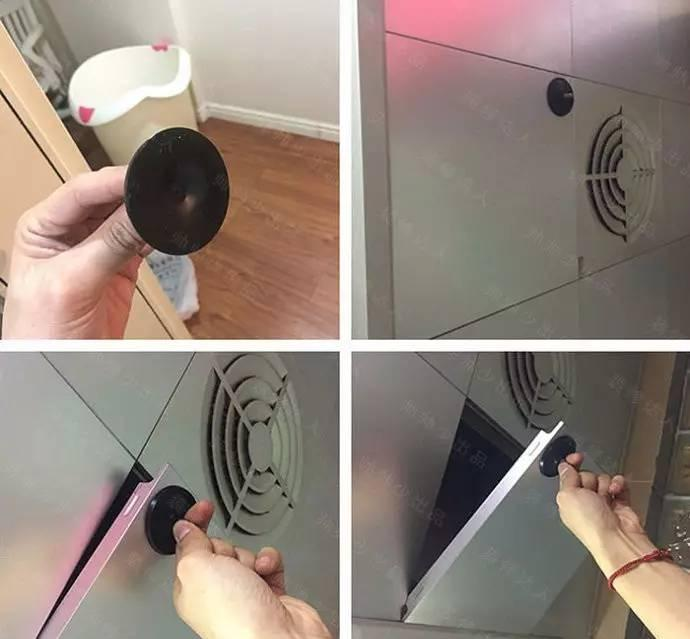|

# 卫生间这六点没做好,以后多的是烦恼(附屋主亲身示范)

有道是“金厨银卫”，一天要进出n遍的卫生间，却也是家里装修坑最多也最容易忽略的区域，今天我们来展开聊聊。

今天分享的一兜糖达人@帅帅少是江浙地区知名的装修红人，四次装修积累许多实战经验，自创“海绵垫”消音法、浴室地面“秒下水”工艺等等，数次被当地报章媒体报道，累计在一兜糖上分享115篇装修干货文章，总阅读量超过20万。帅帅少的浴室柜一直用的都是台上盆，每次洗完头周围一圈水渍，卫生有死角，清理起来异常麻烦，生活经验告诉我们：不要选择台上盆~解决办法有两种： 一更换更大的台上盆；二更换带台下盆的浴室柜。水印康庭入住5年，墙砖的缝隙已经发黑，洗手台上的玻璃胶也已经发黑发霉。

可是你要知道，当初填缝剂用的是国内最好的品牌“牛元”，玻璃胶用的也是新西兰进口的“达尼丁”，可结果呢？只有“真瓷胶”和“美缝剂”才能彻底解决后顾之忧~

真瓷胶填缝以后，会坚硬如瓷，正因如此它不会被灰尘附着，用抹布轻轻一抹就干净了，自然不会发黑发霉。

而相比美缝剂，美缝剂是勾缝剂的升级产品，相比真瓷胶会逊色一些，后期会有脱胶的可能性，价格自然略低于真瓷胶~建议：墙砖使用“美缝剂”填缝，地砖使用“真瓷胶”填缝，有价格预算的土豪可以全部采用真瓷胶！DIY美缝步骤

1、目测

目测首先要从大面积看，看墙体的平整度；

其次看砖与砖之间缝是否对齐了，如果不齐，进一步看是砖有尺差还是工人贴歪了。（建议在考察工人之前就看到他干的活。）

最后要看阳角对缝做的怎么样，太厚了不好看，太薄了，轻轻一碰就掉瓷了。出现这种问题主要原因，一是瓷砖质量不好，二是为了好看整太薄了，所以容易掉瓷。需要叮嘱泥工师傅，不能做太薄。2、检查空鼓率

一般空鼓较多出现在：墙砖的上半部和四角，及阴角铺贴的位置。

空鼓的害处：有空鼓的地方和其它位置伸缩性不一样，时间久了砖容易出现襞缝的情况。有空鼓的地方不受力，在空鼓的地方打孔容易将砖打坏。

检查墙地砖空鼓的办法：在贴砖72小时后，建议用锣丝刀把，将容易出现空鼓的重点位置查找一遍，声音很容易辨别。

建议：墙砖不建议有一点点空鼓就扒下来重贴，在合理范围内别劳民伤财。但发现大面积空鼓的地方一定要扒下来重贴。别怕麻烦。

但地砖有空鼓是绝对不行的，日后容易开裂，有重物碰巧落到空鼓的位置，地砖会立刻破裂。3、检查阴阳角

阴阳角如果达不到90度标准，直接影响到地砖的铺贴和手盆等物品的放置，所以非常重要。

检验起来却很简单，阴角一块砖即可。阳角，两块砖或一块砖+靠尺也可。

4、检查高度差和下水坡度

验收时厨房高度差在2.5公分，外面铺完地板，高度差大约在1公分左右。

卫生间高度差为3.5公分，外面铺完地板，高度差大约在2公分左右。

下水坡度检验可以用水平尺。也可用最简单的办法，倒水。看水是不是往地漏里流就可以了。5、勾缝

一定要提醒工人，别一边干一边勾缝，让砖里面干透，至少要保证48小时后，将缝隙内处理干净，统一勾缝。勾缝剂要用好的，西卡和汉高都是不错的选择~

1、做防水注意的要点

防水一般做到沿墙面30cm高，卫生间淋浴的墙面做到180cm高，如果隔壁墙面有衣柜甚至需要做整面墙壁。

2、实验时间一定要做足

防水实验要做足48小时，而且要保证蓄满水，做防水的那两天要到楼下去看天花板有没有侵湿，如果有重新做，做到合格为止。

目前市场上防水涂料分两种：硬性防水涂料，软性防水涂料。

硬性防水涂料直接刷在水泥浆上面，然后再上面贴砖，防水性能非常好。

软性防水涂料呈液体按比例和在水泥砂浆里面，其防潮、防湿功能非常好。

装修通常用第一种就可以了，在潮湿地区或别墅装修中，帅帅少建议所有装修业主两种方式结合起来使用！有一些户型，卧室的衣柜是紧挨着卫生间的，有一些则更离谱，紧挨着淋浴房。除了卫生间防水，卧室这一边的衣柜也必须要防潮处理。

两件既省钱又好用的法宝：竹炭+防潮纸（防潮膜）

1、衣柜地面铺设竹炭，淘宝上的竹炭包不经济，建议直接购买竹炭或者木炭，袋装的那种，建议家里每个柜子下面都放木炭，木炭不仅可以除湿，而且具有很强的吸附性，对吸收装修后的气味与有害物质有一定功效。2、防潮膜，又称防潮纸，这东西老便宜了，家里铺设地暖、地板的时候都需要用到它，一般都有剩，想要效果好，就在墙壁那一块位置也一起贴上吧，衣柜不要靠墙，留2、3公分的缝隙就好了！* 万一这两步都没做，衣柜却已经装好了，怎么办？

将发泡剂大量打到衣柜和墙体的缝隙中去，不要怕会溢出来，因为发泡剂的特点就是会钻，它会朝着缝隙一直钻，直至填满整个缝隙为止。

集成吊顶很难取下，有了吸盘以后就容易多了。

吸盘挂钩是家里常见的生活用品，将吸盘吸在集成板的某一个角上，一粘一拉，一块集成吊顶就下来了~ 之后换好灯管再把集成板装回去就好了。点击「阅读原文」查看装修达人@帅帅少 的全部分享

`声明：本文由入驻焦点开放平台的作者撰写，除焦点官方账号外，观点仅代表作者本人，不代表焦点立场错误信息举报电话： 400-099-0099，邮箱：jubao@vip.sohu.com，或点此进行意见反馈，或点此进行举报投诉。`A B C D E F G H J K L M N P Q R S T W X Y Z
A - B - C - D - E
• A
• 鞍山
• 安庆
• 安阳
• 安顺
• 安康
• 澳门
• B
• 北京
• 保定
• 包头
• 巴彦淖尔
• 本溪
• 蚌埠
• 亳州
• 滨州
• 北海
• 百色
• 巴中
• 毕节
• 保山
• 宝鸡
• 白银
• 巴州
• C
• 承德
• 沧州
• 长治
• 赤峰
• 朝阳
• 长春
• 常州
• 滁州
• 池州
• 长沙
• 常德
• 郴州
• 潮州
• 崇左
• 重庆
• 成都
• 楚雄
• 昌都
• 慈溪
• 常熟
• D
• 大同
• 大连
• 丹东
• 大庆
• 东营
• 德州
• 东莞
• 德阳
• 达州
• 大理
• 德宏
• 定西
• 儋州
• 东平
• E
• 鄂尔多斯
• 鄂州
• 恩施
F - G - H - I - J
• F
• 抚顺
• 阜新
• 阜阳
• 福州
• 抚州
• 佛山
• 防城港
• G
• 赣州
• 广州
• 桂林
• 贵港
• 广元
• 广安
• 贵阳
• 固原
• H
• 邯郸
• 衡水
• 呼和浩特
• 呼伦贝尔
• 葫芦岛
• 哈尔滨
• 黑河
• 淮安
• 杭州
• 湖州
• 合肥
• 淮南
• 淮北
• 黄山
• 菏泽
• 鹤壁
• 黄石
• 黄冈
• 衡阳
• 怀化
• 惠州
• 河源
• 贺州
• 河池
• 海口
• 红河
• 汉中
• 海东
• 怀来
• I
• J
• 晋中
• 锦州
• 吉林
• 鸡西
• 佳木斯
• 嘉兴
• 金华
• 景德镇
• 九江
• 吉安
• 济南
• 济宁
• 焦作
• 荆门
• 荆州
• 江门
• 揭阳
• 金昌
• 酒泉
• 嘉峪关
K - L - M - N - P
• K
• 开封
• 昆明
• 昆山
• L
• 廊坊
• 临汾
• 辽阳
• 连云港
• 丽水
• 六安
• 龙岩
• 莱芜
• 临沂
• 聊城
• 洛阳
• 漯河
• 娄底
• 柳州
• 来宾
• 泸州
• 乐山
• 六盘水
• 丽江
• 临沧
• 拉萨
• 林芝
• 兰州
• 陇南
• M
• 牡丹江
• 马鞍山
• 茂名
• 梅州
• 绵阳
• 眉山
• N
• 南京
• 南通
• 宁波
• 南平
• 宁德
• 南昌
• 南阳
• 南宁
• 内江
• 南充
• P
• 盘锦
• 莆田
• 平顶山
• 濮阳
• 攀枝花
• 普洱
• 平凉
Q - R - S - T - W
• Q
• 秦皇岛
• 齐齐哈尔
• 衢州
• 泉州
• 青岛
• 清远
• 钦州
• 黔南
• 曲靖
• 庆阳
• R
• 日照
• 日喀则
• S
• 石家庄
• 沈阳
• 双鸭山
• 绥化
• 上海
• 苏州
• 宿迁
• 绍兴
• 宿州
• 三明
• 上饶
• 三门峡
• 商丘
• 十堰
• 随州
• 邵阳
• 韶关
• 深圳
• 汕头
• 汕尾
• 三亚
• 三沙
• 遂宁
• 山南
• 商洛
• 石嘴山
• T
• 天津
• 唐山
• 太原
• 通辽
• 铁岭
• 泰州
• 台州
• 铜陵
• 泰安
• 铜仁
• 铜川
• 天水
• 天门
• W
• 乌海
• 乌兰察布
• 无锡
• 温州
• 芜湖
• 潍坊
• 威海
• 武汉
• 梧州
• 渭南
• 武威
• 吴忠
• 乌鲁木齐
X - Y - Z
• X
• 邢台
• 徐州
• 宣城
• 厦门
• 新乡
• 许昌
• 信阳
• 襄阳
• 孝感
• 咸宁
• 湘潭
• 湘西
• 西双版纳
• 西安
• 咸阳
• 西宁
• 仙桃
• 西昌
• Y
• 运城
• 营口
• 盐城
• 扬州
• 鹰潭
• 宜春
• 烟台
• 宜昌
• 岳阳
• 益阳
• 永州
• 阳江
• 云浮
• 玉林
• 宜宾
• 雅安
• 玉溪
• 延安
• 榆林
• 银川
• Z
• 张家口
• 镇江
• 舟山
• 漳州
• 淄博
• 枣庄
• 郑州
• 周口
• 驻马店
• 株洲
• 张家界
• 珠海
• 湛江
• 肇庆
• 中山
• 自贡
• 资阳
• 遵义
• 昭通
• 张掖
• 中卫

1室1厅1厨1卫1阳台

1
2
3
4
5

0
1
2

1

1

0
1
2
3报名成功，资料已提交审核A B C D E F G H J K L M N P Q R S T W X Y Z
A - B - C - D - E
• A
• 鞍山
• 安庆
• 安阳
• 安顺
• 安康
• 澳门
• B
• 北京
• 保定
• 包头
• 巴彦淖尔
• 本溪
• 蚌埠
• 亳州
• 滨州
• 北海
• 百色
• 巴中
• 毕节
• 保山
• 宝鸡
• 白银
• 巴州
• C
• 承德
• 沧州
• 长治
• 赤峰
• 朝阳
• 长春
• 常州
• 滁州
• 池州
• 长沙
• 常德
• 郴州
• 潮州
• 崇左
• 重庆
• 成都
• 楚雄
• 昌都
• 慈溪
• 常熟
• D
• 大同
• 大连
• 丹东
• 大庆
• 东营
• 德州
• 东莞
• 德阳
• 达州
• 大理
• 德宏
• 定西
• 儋州
• 东平
• E
• 鄂尔多斯
• 鄂州
• 恩施
F - G - H - I - J
• F
• 抚顺
• 阜新
• 阜阳
• 福州
• 抚州
• 佛山
• 防城港
• G
• 赣州
• 广州
• 桂林
• 贵港
• 广元
• 广安
• 贵阳
• 固原
• H
• 邯郸
• 衡水
• 呼和浩特
• 呼伦贝尔
• 葫芦岛
• 哈尔滨
• 黑河
• 淮安
• 杭州
• 湖州
• 合肥
• 淮南
• 淮北
• 黄山
• 菏泽
• 鹤壁
• 黄石
• 黄冈
• 衡阳
• 怀化
• 惠州
• 河源
• 贺州
• 河池
• 海口
• 红河
• 汉中
• 海东
• 怀来
• I
• J
• 晋中
• 锦州
• 吉林
• 鸡西
• 佳木斯
• 嘉兴
• 金华
• 景德镇
• 九江
• 吉安
• 济南
• 济宁
• 焦作
• 荆门
• 荆州
• 江门
• 揭阳
• 金昌
• 酒泉
• 嘉峪关
K - L - M - N - P
• K
• 开封
• 昆明
• 昆山
• L
• 廊坊
• 临汾
• 辽阳
• 连云港
• 丽水
• 六安
• 龙岩
• 莱芜
• 临沂
• 聊城
• 洛阳
• 漯河
• 娄底
• 柳州
• 来宾
• 泸州
• 乐山
• 六盘水
• 丽江
• 临沧
• 拉萨
• 林芝
• 兰州
• 陇南
• M
• 牡丹江
• 马鞍山
• 茂名
• 梅州
• 绵阳
• 眉山
• N
• 南京
• 南通
• 宁波
• 南平
• 宁德
• 南昌
• 南阳
• 南宁
• 内江
• 南充
• P
• 盘锦
• 莆田
• 平顶山
• 濮阳
• 攀枝花
• 普洱
• 平凉
Q - R - S - T - W
• Q
• 秦皇岛
• 齐齐哈尔
• 衢州
• 泉州
• 青岛
• 清远
• 钦州
• 黔南
• 曲靖
• 庆阳
• R
• 日照
• 日喀则
• S
• 石家庄
• 沈阳
• 双鸭山
• 绥化
• 上海
• 苏州
• 宿迁
• 绍兴
• 宿州
• 三明
• 上饶
• 三门峡
• 商丘
• 十堰
• 随州
• 邵阳
• 韶关
• 深圳
• 汕头
• 汕尾
• 三亚
• 三沙
• 遂宁
• 山南
• 商洛
• 石嘴山
• T
• 天津
• 唐山
• 太原
• 通辽
• 铁岭
• 泰州
• 台州
• 铜陵
• 泰安
• 铜仁
• 铜川
• 天水
• 天门
• W
• 乌海
• 乌兰察布
• 无锡
• 温州
• 芜湖
• 潍坊
• 威海
• 武汉
• 梧州
• 渭南
• 武威
• 吴忠
• 乌鲁木齐
X - Y - Z
• X
• 邢台
• 徐州
• 宣城
• 厦门
• 新乡
• 许昌
• 信阳
• 襄阳
• 孝感
• 咸宁
• 湘潭
• 湘西
• 西双版纳
• 西安
• 咸阳
• 西宁
• 仙桃
• 西昌
• Y
• 运城
• 营口
• 盐城
• 扬州
• 鹰潭
• 宜春
• 烟台
• 宜昌
• 岳阳
• 益阳
• 永州
• 阳江
• 云浮
• 玉林
• 宜宾
• 雅安
• 玉溪
• 延安
• 榆林
• 银川
• Z
• 张家口
• 镇江
• 舟山
• 漳州
• 淄博
• 枣庄
• 郑州
• 周口
• 驻马店
• 株洲
• 张家界
• 珠海
• 湛江
• 肇庆
• 中山
• 自贡
• 资阳
• 遵义
• 昭通
• 张掖
• 中卫• 手机• 分享
• 设计
免费设计
• 计算器
装修计算器
• 入驻
合作入驻
• 联系
联系我们
• 置顶
返回顶部